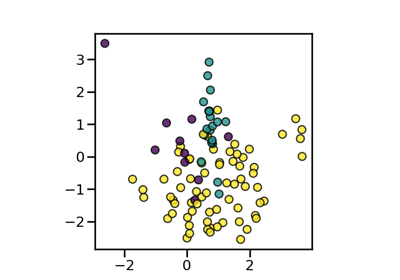# imblearn.combine.SMOTEENN¶

class imblearn.combine.SMOTEENN(*, sampling_strategy='auto', random_state=None, smote=None, enn=None, n_jobs=None)[source]

Over-sampling using SMOTE and cleaning using ENN.

Combine over- and under-sampling using SMOTE and Edited Nearest Neighbours.

Read more in the User Guide.

Parameters
sampling_strategyfloat, str, dict or callable, default=’auto’

Sampling information to resample the data set.

• When float, it corresponds to the desired ratio of the number of samples in the minority class over the number of samples in the majority class after resampling. Therefore, the ratio is expressed aswhereis the number of samples in the minority class after resampling andis the number of samples in the majority class.

Warning

float is only available for binary classification. An error is raised for multi-class classification.

• When str, specify the class targeted by the resampling. The number of samples in the different classes will be equalized. Possible choices are:

'minority': resample only the minority class;

'not minority': resample all classes but the minority class;

'not majority': resample all classes but the majority class;

'all': resample all classes;

'auto': equivalent to 'not majority'.

• When dict, the keys correspond to the targeted classes. The values correspond to the desired number of samples for each targeted class.

• When callable, function taking y and returns a dict. The keys correspond to the targeted classes. The values correspond to the desired number of samples for each class.

random_stateint, RandomState instance, default=None

Control the randomization of the algorithm.

• If int, random_state is the seed used by the random number generator;

• If RandomState instance, random_state is the random number generator;

• If None, the random number generator is the RandomState instance used by np.random.

smoteobject, default=None

The imblearn.over_sampling.SMOTE object to use. If not given, a imblearn.over_sampling.SMOTE object with default parameters will be given.

ennobject, default=None

The imblearn.under_sampling.EditedNearestNeighbours object to use. If not given, a imblearn.under_sampling.EditedNearestNeighbours object with sampling strategy=’all’ will be given.

n_jobsint, default=None

Number of CPU cores used during the cross-validation loop. None means 1 unless in a joblib.parallel_backend context. -1 means using all processors. See Glossary for more details.

SMOTETomek

Over-sample using SMOTE followed by under-sampling removing the Tomek’s links.

Notes

The method is presented in .

Supports multi-class resampling. Refer to SMOTE and ENN regarding the scheme which used.

References

1

G. Batista, R. C. Prati, M. C. Monard. “A study of the behavior of several methods for balancing machine learning training data,” ACM Sigkdd Explorations Newsletter 6 (1), 20-29, 2004.

Examples

>>> from collections import Counter
>>> from sklearn.datasets import make_classification
>>> from imblearn.combine import SMOTEENN
>>> X, y = make_classification(n_classes=2, class_sep=2,
... weights=[0.1, 0.9], n_informative=3, n_redundant=1, flip_y=0,
... n_features=20, n_clusters_per_class=1, n_samples=1000, random_state=10)
>>> print('Original dataset shape %s' % Counter(y))
Original dataset shape Counter({1: 900, 0: 100})
>>> sme = SMOTEENN(random_state=42)
>>> X_res, y_res = sme.fit_resample(X, y)
>>> print('Resampled dataset shape %s' % Counter(y_res))
Resampled dataset shape Counter({0: 900, 1: 881})

__init__(self, *, sampling_strategy='auto', random_state=None, smote=None, enn=None, n_jobs=None)[source]

Initialize self. See help(type(self)) for accurate signature.

## Examples using imblearn.combine.SMOTEENN¶